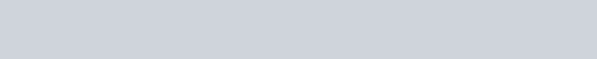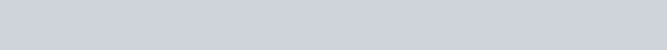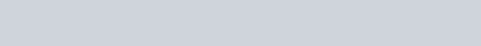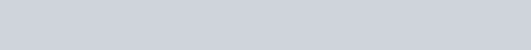# Fixed Bias Configuration with a Divider Resistor in the Base Complete Analysis

This article will uncover the fixed bias configuration with a divider resistor complete analysis. Actually this is another variation of a fixed bias circuit as shown in Figure 87. The only difference of this circuit is the addition of a resistor RB2 as shown in the figure. At application wherein the base is not tied up with the supply , when the supply to the base will float (no define logic low state to turn off the device), RB2 resistor  do not let it to happen by pulling the base to the ground and hence preventing a possible noise initiated triggering or simply a false triggering.

Figure 88 shows a fixed bias with base divider that is designed only to operate with DC application. When  cannot provide a sure logic low to drive the transistor into cut-off, resistor will pull the base down. With this, the base will not float and it will not be triggered by noise or false triggering will be avoided.Figure 87 – Fixed bias circuit with a base divider resistor designed for ac application. This is the same to the fixed bias; it cannot provide stability against beta variationFigure 88 – Variation of Figure 87 which is only designed for DC operation. This configuration is often used for switch operation such as relay driver and other logic circuits. The presence of R_B2 prevents the base to float and therefore provide the circuit with noise immunity when there is no defined logic low from V_BB.

Base-Emitter Analysis

We will start the analysis by applying KCL at node A of Figure 88. Derivation results are also applicable to Figure 87 but you need to change VBB with VCC.

We are going to assume current entering the node with a positive sign and negative for current leaving it.The voltage across the resistor RB2 is equal to the  VBE of the transistor since they are directly parallel. Hence, the current  isThe current IB1 isNow, the base current isThen, we will substitute the individual current expression will giveCollector-Emitter Analysis

The analysis of the collector-emitter circuit is the same with the previous configuration. Let’s start by doing a KVL from the  VCC down to the circuit ground.Expressing  VCE will giveOperation at Saturation

At saturation, the voltage drop in the collector-emitter is ideally zero or equal to the saturation voltage of the transistor. The collector current is no longer dependent of the base current and the amplification factor of the device. It can be written asExample 24

For the circuit in Figure 90, compute the following:

a) Base current b) Collector current c) Vc d) VBC e) Power dissipation f) Assuming the circuit saturates, compute for the collector current.Figure 90 – Circuit for Example 24. VCC=10V, VBB=8V, RB1=100kΩ, RB2=10kΩ, RC=2kΩ, VBE=0.6V and β=100.

Solution

a) Solving base current using [Eq. 131]

VC is the voltage measured in the collector with respect to the circuit ground. It can be expressed from the definition of VCE.VE is the emitter voltage and since the circuit do not have an emitter resistor, it is equal to zero thus making VC=VCE.

Solve for  VCE using [Eq. 132]So the value of  VC is also 7.2V.

d) Solve for VBCSince VE=0, so VB=VBE, and the value of VBC isThe negative sign means that the base-collector junction is reversed biased which is expected since the circuit operates in the linear mode.

e) Solve for power dissipationSince the saturation voltage of the device is not given, in the above computation we assumed it to be zero ideally.

The addition of a pull down resistor in a fixed bias configuration with a divider resistor is because of the need to pull down the base in case the base applied voltage will float. Allowing the base to float is not a good idea since noise can falsely trigger it.

This site uses Akismet to reduce spam. Learn how your comment data is processed.